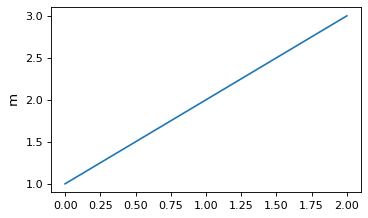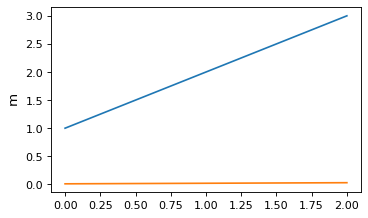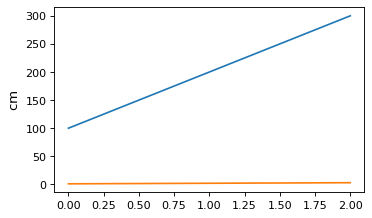# Plotting Astropy objects in Matplotlib¶

## Plotting quantities¶

`Quantity` objects can be conveniently plotted using matplotlib. This feature needs to be explicitly turned on:

```>>> from astropy.visualization import quantity_support
>>> quantity_support()
<astropy.visualization.units.MplQuantityConverter ...>
```

Then `Quantity` objects can be passed to matplotlib plotting functions. The axis labels are automatically labeled with the unit of the quantity:

```from astropy import units as u
from astropy.visualization import quantity_support
quantity_support()
from matplotlib import pyplot as plt
plt.figure(figsize=(5,3))
plt.plot([1, 2, 3] * u.m)
```

(png, svg, pdf)Quantities are automatically converted to the first unit set on a particular axis, so in the following, the y-axis remains in `m` even though the second line is given in `cm`:

```plt.plot([1, 2, 3] * u.cm)
```

(png, svg, pdf)Plotting a quantity with an incompatible unit will raise an exception. For example, calling `plt.plot([1, 2, 3] * u.kg)` (mass unit) to overplot on the plot above that is displaying length units.

To make sure unit support is turned off afterward, you can use `quantity_support` with a `with` statement:

```with quantity_support():
plt.plot([1, 2, 3] * u.m)
```

## Plotting times¶

Matplotlib natively provides a mechanism for plotting dates and times on one or both of the axes, as described in Date tick labels. To make use of this, you can use the `plot_date` attribute of `Time` to get values in the time system used by Matplotlib.

However, in many cases, you will probably want to have more control over the precise scale and format to use for the tick labels, in which case you can make use of the `time_support` function. This feature needs to be explicitly turned on:

```>>> from astropy.visualization import time_support
>>> time_support()
<astropy.visualization.units.MplTimeConverter ...>
```

Once this is enabled, `Time` objects can be passed to matplotlib plotting functions. The axis labels are then automatically labeled with times formatted using the `Time` class:

```from matplotlib import pyplot as plt
from astropy.time import Time
from astropy.visualization import time_support

time_support()

plt.figure(figsize=(5,3))
plt.plot(Time([58000, 59000, 62000], format='mjd'), [1.2, 3.3, 2.3])
```

(png, svg, pdf)By default, the format and scale used for the plots is taken from the first time that Matplotlib encounters for a particular Axes instance. The format and scale can also be explicitly controlled by passing arguments to `time_support`:

```time_support(format='mjd', scale='tai')
plt.figure(figsize=(5,3))
plt.plot(Time([50000, 52000, 54000], format='mjd'), [1.2, 3.3, 2.3])
```

(png, svg, pdf)To make sure support for plotting times is turned off afterward, you can use `time_support` as a context manager:

```with time_support(format='mjd', scale='tai'):
plt.figure(figsize=(5,3))
plt.plot(Time([50000, 52000, 54000], format='mjd'))
```